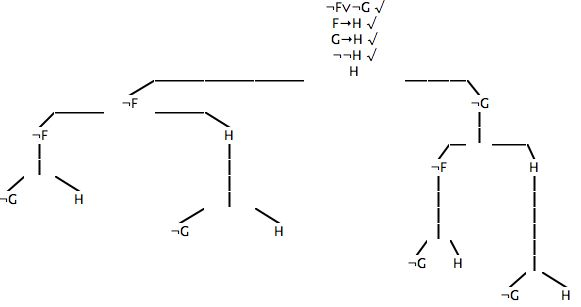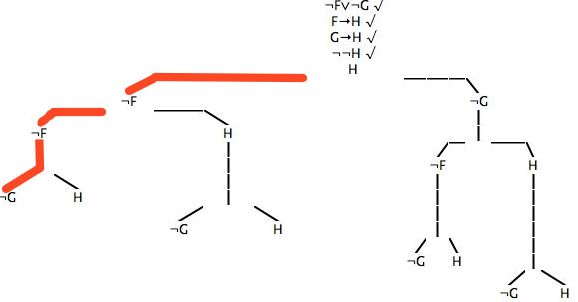# Tree Tutorial 3: Using Trees to Test for Satisfiability and Invalidity

Logical System

2013

### Skills to be acquired in this tutorial:

To become familiar with the notions of closed and complete trees. To be able to use trees to test for satisfiability and invalidity.

Colin Howson,  Logic with trees Chapter 2

### Tutorial:

#### Closure and completeness

In Tutorial 1, we met the notions of closed and open branches (a closed branch was one containing a formula and also the negation of that formula, an open branch was a branch that was not closed).

These concepts can be extended to trees. A closed tree is a tree in which every branch is closed. An open tree is a tree which has at least one open branch.

When a tree growing rule is applied to a formula in the tree, that formula is considered dead. [It is ticked and never used again.] The formulas that result from a single application of a tree growing rule are themselves shorter than the original formula. This means that with sentential tree growing, for a single formula or collection of formulas, growth must eventually stop. All sentential trees are of finite size. They may grow for a while, but eventually that growth must stop. When all the growth is finished, a tree is complete.

There are some facts about closed trees and complete trees, which we will use (but not prove here).

• If a tree is closed, the original formulas of the root are not simultaneously satisfiable ie there no assignment of truth values to the atomic components under which the formulas of the root all come out to be true.
• If a complete tree has an open branch, the original formulas of the root are simultaneously satisfiable ie there is an assignment of truth values to the atomic components under which the formulas of the root all come out to be true.

These facts can be put to use immediately. First they can be used to tell whether a collection of formulas is satisfiable. For example, is

F ∧ G, G→H,¬H∨¬GAnother example, is

F ∧ G, G→H,¬H∨GSecond, this technique and process can be used to test the validity of arguments. An argument is invalid if, and only if, it is possible for all its premises to be true and its conclusion false, at one and the same time. That is, an argument is invalid if, and only if, it all its premises and the negation of its conclusion are (simultaneously) satisfiable. So, if a tree is grown from the premises of an argument, together with the negation of its conclusion:- if that tree is closed, the argument is valid; if that tree is complete and open, the argument is invalid.

Two examples, is the argument F∨G, F→H,G→H∴H valid? Answer: YesIs the argument ¬F∨¬G, F→H,G→H∴¬H valid? Answer: NoThe tree technique has another very useful feature. If the root formulas are satisfiable, it allows you to construct a valuation that will satisfy them.

All you do is to choose any open branch. SayThen make assignments to the atomic formulas and negations of atomic formulas, as follows:-

if <atomic formula> appears in the branch, assign <atomic formula> true
if ¬<atomic formula> appears in the branch, assign <atomic formula> false

Using this on the example, F is assigned false, G is assigned false, and H is assigned true. This gives us, for the root formulas

¬false∨¬false,
false→true,
false→true
¬¬true

which is

true∨true,
true,
true
¬false

which is

true,
true,
true
true

ie all the root formulas are true.

## Exercises to accompany Tree Tutorial 3

### Exercise 1 (of 5):

Show each of the following arguments to be valid (ie produce closed trees for them)

a) F∨G ∴G∨F
b) (Z∧W)→(L∨K),(W∧Z)∴K∨L
c) ¬Y→¬Z,¬Z→¬X,¬X→¬Y∴Y↔Z

### Exercise 2 (of 5):

Determine whether each of the following statements is a tautology. [A tautology is a logical truth; that is, it is always true no matter what the assignment; that is, it is not possible for it to be false; that is, it is not possible for its negation to be true; that is, a complete tree for its negation will be closed. So the test is: a complete tree for its negation will not have an open branch.]

a) (F↔G)↔(G↔F)
b) (F→G)↔(G↔F)
c) P ∨¬P

### Exercise 3 (of 5):

Determine whether each of the following statements is a contradiction. [It is not possible for contradiction to be true; that is, a complete tree for it will be closed. So the test is: a complete tree for it will not have an open branch.]

a) P ∧¬P
b) (((Z∧W)→(L∨K))∧(W∧Z))∧¬(K∨L)
c) F→¬F

### Exercise 4 (of 5):

Determine whether each of the following statements is a consistent. [It must be possible for consistent statement to be true; that is, a complete tree for it will be open. So the test is: a complete tree for it will have an open branch.]

a) P ∧¬P
b) (((Z∧W)→(L∨K))∧(W∧Z))∧¬(K∨L)
c) F→¬F